# Samacheer Kalvi 8th Maths Solutions Term 1 Chapter 4 Geometry Ex 4.3

Students can Download Maths Chapter 4 Geometry Ex 4.3 Questions and Answers, Notes Pdf, Samacheer Kalvi 8th Maths Book Solutions Guide Pdf helps you to revise the complete Tamilnadu State Board New Syllabus and score more marks in your examinations.## Tamilnadu Samacheer Kalvi 8th Maths Solutions Term 1 Chapter 4 Geometry Additional Questions

Construct the quadrilaterals with the following measurements and also find their area.

Question 1.
ABCD, AB = 5 cm, BC 4.5 cm, CD = 3.8 cm, DA = 4.4 cm and AC = 6.2 cm.
Solution:
Given AB = 5 cm,
BC = 4.5 cm,
CD = 3.8 cm,
DA = 4.4 cm,
AC = 6.2 cm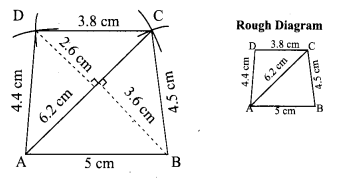Steps:
1. Draw a line segment AB = 5 cm
2. With A and B as centers drawn arcs of radii 6.2 cm and 4.5cm respectively and let them cut at C.
3. Joined AC and BC.
4. With A and C as centrers drawn arcs of radii 4.4cm and 3.8 cm respectively and let them at D.
6. ABCD is the required quadrilateral.
Calculation of Area: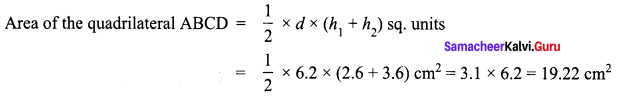Question 2.
KITE, KI = 5.4 cm, IT = 4.6 cm, TE= 4.5 cm, KE = 4.8 cm and IE = 6 cm.
Solution:
Given, KI = 5.4 cm,
IT = 4.6 cm,
TE= 4.5 cm,
KE = 4.8 cm,
IE = 6 cm.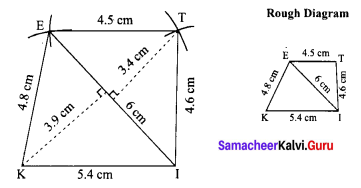Steps:
1. Draw a line segment KI = 5.4 cm
2. With K and I as centers drawn arcs of radii 4.8 cm and 6 cm respectively and let them cut at E.
3. Joined KE and IE.
4. With E and I as centers, drawn arcs of radius 4.5cm and 4.6 cm respectively and let them cut at T.
5. Joined ET and IT.
6. KITE is the required quadrilateral.
Calculation of Area: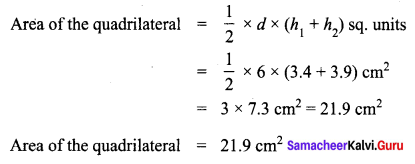Question 3.
PLAY, PL = 7 cm, LA = 6 cm, AY= 6 cm, PA = 8 cm and LY = 7 cm.
Solution:
Given PL = 7 cm,
LA = 6 cm,
AY= 6 cm,
PA = 8 cm,
LY = 7 cm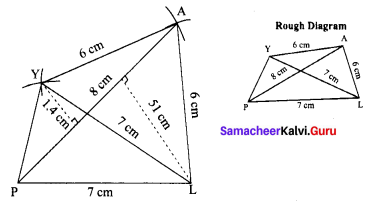Steps:
1. Draw a line segment PL = 7 cm
2. With P and L as centers, drawn arcs of radii 8 cm and 6 cm respectively, let them cut at A.
3. Joined PA and LA.
4. With L and A as centers, drawn arcs of radii 7 cm and 6 cm respectively and let them cut at Y.
5. Joined LY, PY and AY.
6. PLAY is the required quadrilateral.
Calculation of Area: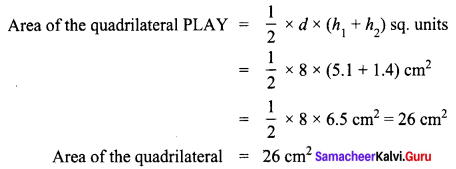Question 4.
LIKE, LI = 4.2 cm, IK = 7 cm, KE = 5 cm, LK = 6 cm and IE = 8 cm.
Solution:
LI = 4.2 cm,
IK = 7 cm,
KE = 5 cm,
LK = 6 cm,
IE = 8 cm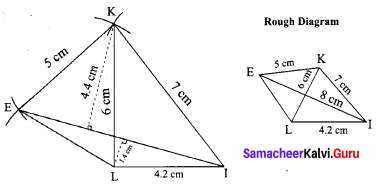Steps:
1. Draw a line segment LI = 4.2 cm
2. With L and I as centers, drawn arcs of radii 6 cm and 7 cm respectively and let them cut at K.
3. Joined LK and IK.
4. With I and K as centers, drawn arcs of radius 8 cm and 5 cm respectively and let them cut at E.
5. Joined LE, IE and KE.
6. LIKE is the required quadrilateral.
Calculation of Area: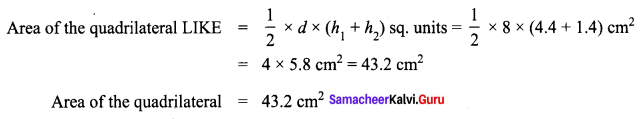Question 5.
PQRS, PQ = QR = 3.5 cm, RS = 5.2 cm, SP = 5.3 cm and ∠Q =120° .
Solution:
PQ = QR = 3.5 cm,
RS = 5.2 cm,
SP = 5.3 cm ,
∠Q =120°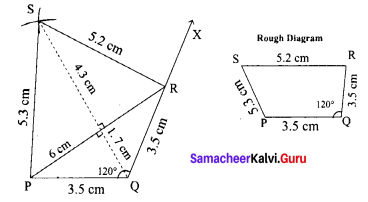Steps:
1. Draw a line segment PQ = 3.5 cm
2. Made ∠Q = 120°. Drawn the ray QX.
3. With Q as centre drawn an arc of radius 3.5 cm. Let it cut the ray QX at R.
4. With R and P as centres drawn arcs of radii 5.2cm and 5.5 cm respectively and let them cut at S.
5. Joined PS and RS.
6. PQRS is the required quadrilateral.
Calculation of Area: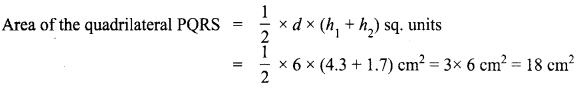Area of the quadrilateral PQRS = 18 cm2

Question 6.
EASY, EA = 6 cm, AS = 4 cm, SY = 5 cm, EY = 4.5 cm and ∠E = 90°.
Solution:
EA = 6 cm,
AS = 4 cm,
SY = 5 cm,
EY = 4.5 cm,
∠E = 90°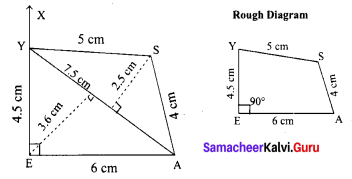1. Drawn a line segment EA = 6 cm
2. Made ∠E = 90°. From E drawn the ray EX.
3. With E as center drawn an arc of 4.5 cm radius. Let of cut the ray EX at Y.
4. With A and Y as centres drawn arcs of radii 4 cm and 5 cm respectively and let them cut at S.
5. Joined AS and YS.
6. EASY is the required quadrilateral.
Calculation of Area: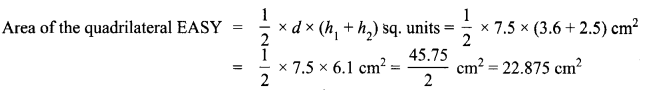∴ Area of the quadrilateral = 22.87 cm2Question 7.
MIND, MI = 3.6 cm, ND = 4 cm, MD = 4 cm, ∠M = 50° and ∠D = 100°.
Solution:
MI = 3.6 cm,
ND = 4 cm,
MD= 4 cm,
∠M = 50°,
∠D = 100°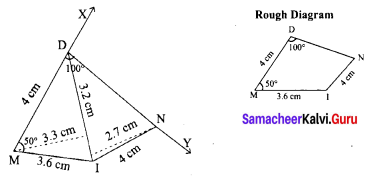1. Draw a line segment MI = 3.6 cm
2. At M on MI made an angle ∠IMX = 50°
3. Drawn an arc with center M and radius 4 cm let it cut MX it D
4. At D on DM made an angle ∠MDY = 100°
5. With I as center drawn an arc of radius 4 cm, let it cut DY at N.
6. Joined DN and IN.
7. MIND is the required quadrilateral.
Calculation of Area: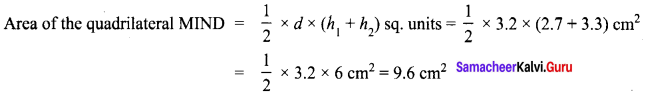Area of the quadrilateral = 9.6 cm2

Question 8.
WORK, WO = 9 cm, OR = 6 cm, RK = 5 cm, ∠O = 100° and ∠R = 60°.
Solution:
WO = 9 cm,
OR = 6 cm,
RK = 5 cm,
∠O = 100°,
∠R = 60°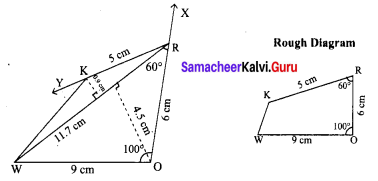Steps:
1. Drawn a line segment WO = 9 cm
2. At O on WO made an angle ∠WOR = 100° and drawn the ray OX.
3. Drawn an arc of radius 6 cm with center O. Let it intersect OX at R.
4. At R on OR, made ∠ORY = 60°, and drawn the ray RY.
5. With center R drawn an arc of radius 5 cm, let it intersect RY at K.
6. Joined WK.
7. WORK is the required quadrilateral.
Calculation of Area: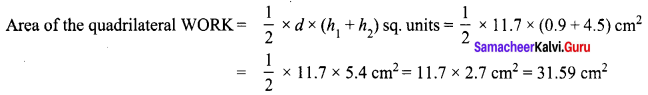Area of the quadrilateral = 31.59 cm2

Question 9.
AGRI, AG = 4.5 cm, GR = 3.8 cm, ∠A = 90°, ∠G = 110° and ∠R = 90°.
Solution:
AG = 4.5 cm,
GR = 3.8 cm,
∠A = 90°,
∠G = 110°,
∠R = 90°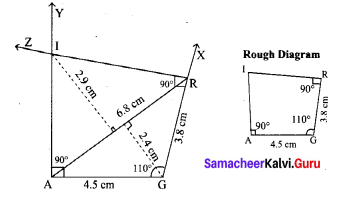1. Draw a line segment AG = 4.5 cm
2. At G on AG made ∠AGX =110°
3. With G as centre drawn an arc of radius 3.8 cm let it cut GX at R.
4. At R on GR made ∠GRZ = 90°
5. At A on AG made ∠GAY = 90°
6. AY and RZ meet at I.
7. AGRI is the required quadrilateral.
Calculation of Area: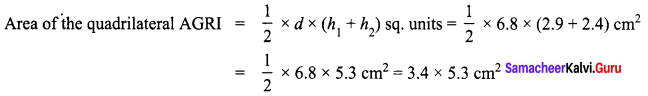Question 10.
YOGA, YO = 6 cm, OG = 6 cm, ∠O = 55°, ∠G = 55° and ∠A = 55°.
Solution:
YO = 6 cm,
OG = 6 cm,
∠O = 55°,
∠G = 55°,
∠A = 55°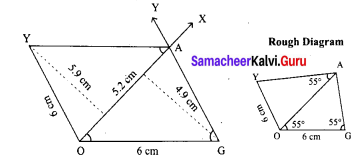Steps:
1. Draw a line segment OG = 6 cm
2. At G on DG made an angle ∠OGY = 55°
3. AT G on GO made ∠GOX = 55°.
4. GY and OX meet cut A.
5. At A on OA made ∠OAZ = 55°
6. Drawn an arc of radius 6 cm with center O. It cut AZ at Y. Joined OY.
7. YOGA is the required quadrilateral.
Calculation of Area: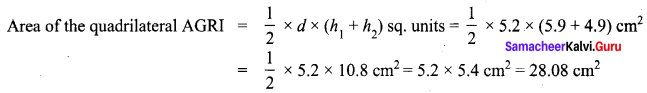Area of the quadrilateral YOGA = 28.08 cm2